# Colleges with the lowest SAT scores in California

Top 10 colleges in California with the lowest SAT scores
Looking for the colleges with the lowest SAT scores in California? Well you're in luck! We've compiled a national college database and have created a list of the top 10 universities with the lowest SAT scores in California below. If you are not a good test taker or worried about your test scores, this list is for you. These are the schools whose applicants had the lowest average SAT scores in California, which means that you can get into these colleges with a lower SAT score. We also include each college's ACT scores and acceptance rate so that you can see where you would have the easiest time getting in. Read on to find out more.

## California State University Dominguez Hills SAT scores

The average SAT score for California State University Dominguez Hills is 900.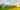The average SAT score of 900 breaks down into:

• SAT math: 440

The average ACT score for California State University Dominguez Hills is 18 and their acceptance rate is 77.2%.

## Holy Names University SAT scores

The average SAT score for Holy Names University is 950.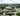The average SAT score of 950 breaks down into:

• SAT math: 470

The average ACT score for Holy Names University is 20 and their acceptance rate is 52.6%.

## Ashford University SAT scores

The average SAT score for Ashford University is 970.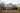The average SAT score of 970 breaks down into:

• SAT math: 485

The average ACT score for Ashford University is 18 and their acceptance rate is 100%.

## California State University Los Angeles SAT scores

The average SAT score for California State University Los Angeles is 985.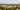The average SAT score of 985 breaks down into:

• SAT math: 490

The average ACT score for California State University Los Angeles is 18 and their acceptance rate is 41.5%.

## California State University East Bay SAT scores

The average SAT score for California State University East Bay is 992.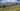The average SAT score of 992 breaks down into:

• SAT math: 490

The average ACT score for California State University East Bay is 19 and their acceptance rate is 71.6%.

## California State University Bakersfield SAT scores

The average SAT score for California State University Bakersfield is 993.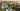The average SAT score of 993 breaks down into:

• SAT math: 490

The average ACT score for California State University Bakersfield is 19 and their acceptance rate is 100%.

## California State University San Bernardino SAT scores

The average SAT score for California State University San Bernardino is 1000.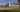The average SAT score of 1000 breaks down into:

• SAT math: 500

The average ACT score for California State University San Bernardino is 18 and their acceptance rate is 54.8%.

## California State University Stanislaus SAT scores

The average SAT score for California State University Stanislaus is 1000.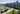The average SAT score of 1000 breaks down into:

• SAT math: 490

The average ACT score for California State University Stanislaus is 19 and their acceptance rate is 85.8%.

## California State Fresno SAT scores

The average SAT score for California State Fresno is 1010.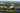The average SAT score of 1010 breaks down into:

• SAT math: 500

The average ACT score for California State Fresno is 19 and their acceptance rate is 57.5%.

## Mount Saint Mary's College SAT scores

The average SAT score for Mount Saint Mary's College is 1020.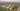The average SAT score of 1020 breaks down into:

• SAT math: 500